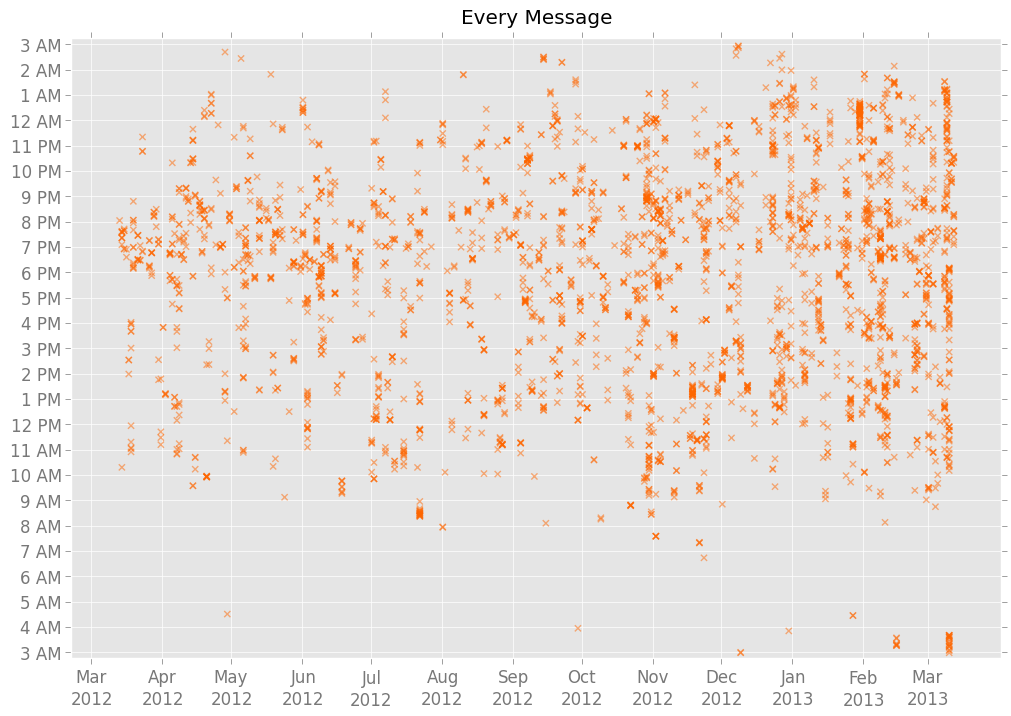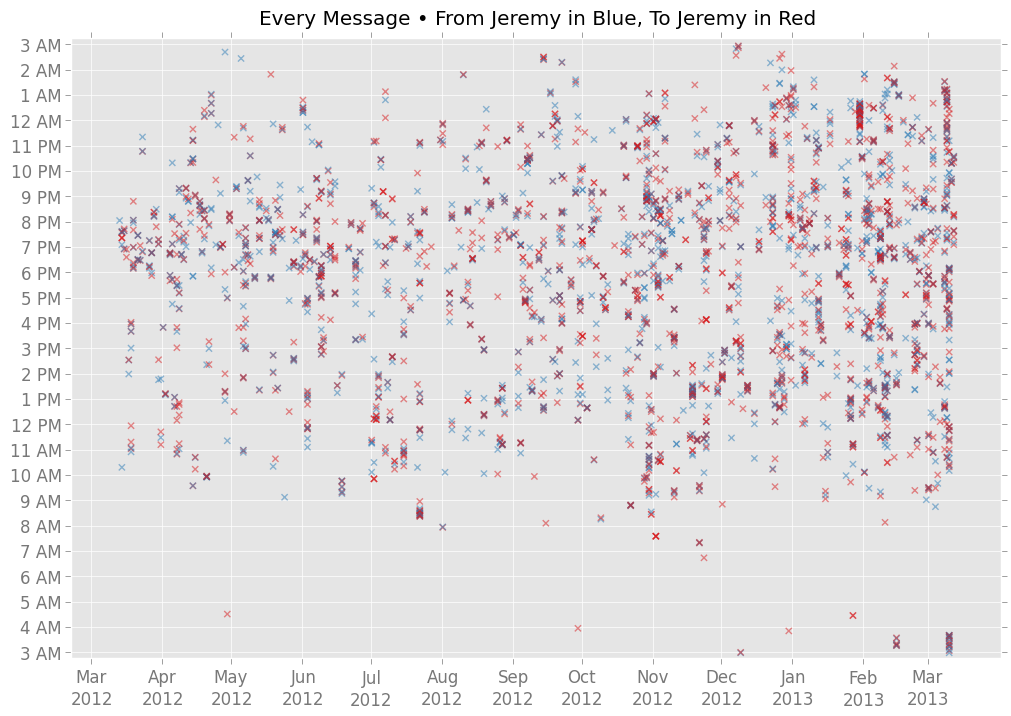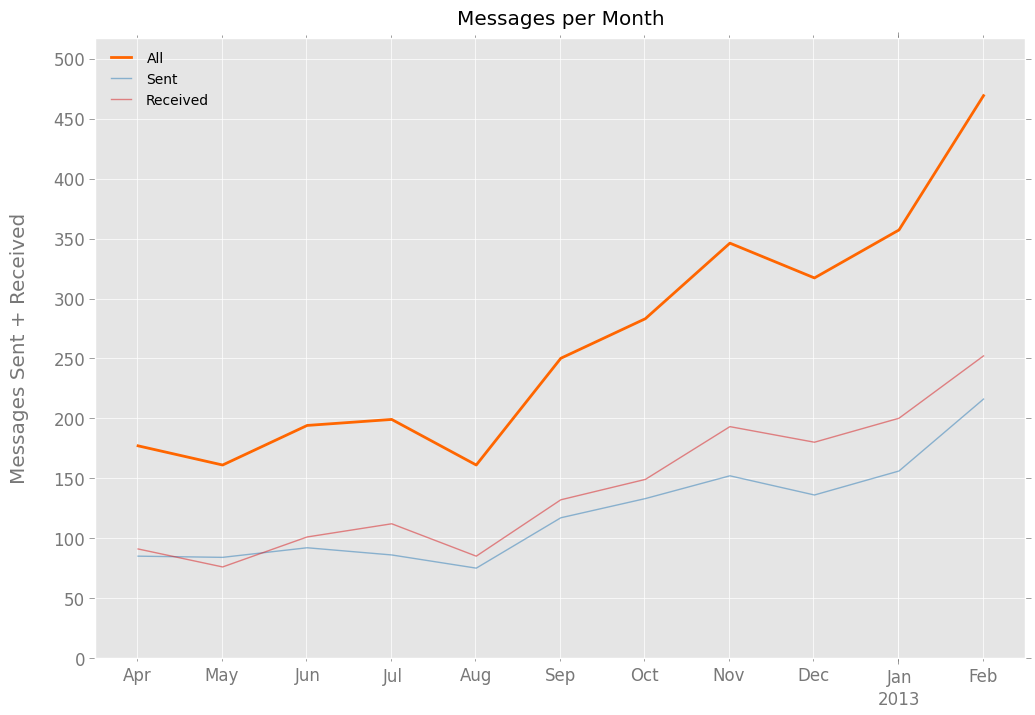# Setup!¶

In :
%pylab inline
%reset

Welcome to pylab, a matplotlib-based Python environment [backend: module://IPython.zmq.pylab.backend_inline].

In :
import datetime
import numpy as np
import pandas as pd
import matplotlib as mpl
import sys, os, re

In :
# Directory helpers
BASE_DIR = os.getcwd()
CHART_DIR = os.path.join(BASE_DIR, "charts")

In :
# Some me-specific constants
SMS_FILE = "../backups/sms-20130317190417.csv"
MY_NAME = "Jeremy"

In :
# Ultimately, let's consider 3am, instead of midnight, to be the end of the day.
HOUR_SHIFT = 3

In :
# Colors via http://colorbrewer2.com/
WHITE, LIGHT_GRAY, GRAY, BLACK = [ "#FFFFFF", "#E5E5E5", "#777777", "#000000" ]
COLORS = [ "#FF6600", "#D7191C", "#2C7BB6", "#FFFFBF", "#ABD9E9" ]
ORANGE, RED, BLUE, YELLOW, LIGHT_BLUE = COLORS
HIGH_ALPHA = 0.9
MEDIUM_ALPHA = 0.5
LOW_ALPHA = 0.1
rgba = mpl.colors.colorConverter.to_rgba

In :
def set_styles(style_dict):
"""Set matplotlib styles from nested a nested dictionary"""
for obj in style_dict: mpl.rc(obj, **style_dict[obj])

# Styles mostly derived from https://github.com/tonysyu/mpltools/blob/master/mpltools/style/ggplot.rc
set_styles({
"figure": { "figsize": [ 12, 8 ], "facecolor": WHITE },
"savefig": { "dpi": 100, "bbox": "tight" },
"patch": { "linewidth": 0.5, "facecolor": ORANGE, "edgecolor": WHITE, "antialiased": True },
"font": { "size": 12 },
"legend": { "fontsize": 10 },
"axes": {
"facecolor": LIGHT_GRAY,
"edgecolor": WHITE,
"linewidth": 1,
"grid": True,
"titlesize": "large",
"labelsize": "large",
"labelcolor": GRAY,
"axisbelow": True,
"color_cycle": COLORS
},
"xtick": { "color": GRAY, "direction": "out" },
"ytick": { "color": GRAY, "direction": "out" },
"grid": { "color": WHITE, "linestyle": "-" }
})

In :
def savechart(filename):
"""Save currently open chart as a PNG to the chart directory."""
mpl.pyplot.savefig(os.path.join(CHART_DIR, filename + ".png"), bbox_inches='tight')

close = mpl.pyplot.close

def humancount(x):
"""Abbreviate a large number into something more readable."""
if x == 0: return "0"
magnitude = int(np.log10(x))
thousands = magnitude / 3
divisor = pow(1000, thousands)
divided = float(x)/divisor
abbvs = [ "", "k", "m" ]
return ((("%." + str(thousands) + "f") if divided % 1 > 0 else "%d") % (divided)) + abbvs[thousands]

def get_step(max_val):
"""Given a max value, return a good step size for y-axis tick marks"""
magnitude = int(np.log10(max_val))
step = pow(10, magnitude - 1)
return step if max_val / step < 25 else step * 5

"""Add a little extra space to the left and right of a date-based x-axis."""
old = axis.get_view_interval()
axis.set_view_interval(old - 0.5, old + 0.5)


# The Data!¶

### Read in CSV of all messages¶

In :
COLS = ["contact_name", "date", "body", "type" ]


### Filter out messages to/from people not in my contacts¶

In :
msgs = msgs[msgs["contact_name"] != "(Unknown)"]


### Filter out unsent messages¶

"type" values:

• 2: sent
• 3: draft
• 4: ???
• 5: failed/error
In :
msgs = msgs[msgs["type"] < 3]


### Add Pythonic datetime, and restrict data to one year from start¶

In :
msgs["datetime"] = msgs["date"].apply(lambda x: datetime.datetime.fromtimestamp(x / 1000))

FIRST_TIMESTAMP = msgs["datetime"].iget(0)
LAST_TIMESTAMP = FIRST_TIMESTAMP + datetime.timedelta(days=365)
msgs = msgs[msgs["datetime"] < LAST_TIMESTAMP]


### Add columns for different measures of time¶

In :
# Hour of day -- 12am as 0, 1am as 1, ..., 1pm as 13, ..., 11pm as 23
msgs["hour"] = msgs["datetime"].apply(lambda x: x.hour)

# Day of week -- with Monday as 0, Tuesday as 1...
msgs["weekday"] = msgs["datetime"].apply(lambda x: x.weekday())

# Most recent Monday
msgs["week"] = pd.DatetimeIndex(msgs["datetime"].apply(lambda x: x.date() - datetime.timedelta(days=x.weekday())))

# First day of month
msgs["month"] = pd.DatetimeIndex(msgs["datetime"].apply(lambda x: pd.Period(x, freq="M").to_timestamp()))


### Calculate some basic things about the range of dates included¶

In :
DATE_RANGE, WEEK_RANGE, MONTH_RANGE = (pd.period_range(FIRST_TIMESTAMP, LAST_TIMESTAMP, freq=x) for x in ("D", "W-MON", "M"))


Adding the message multiplier ("160s") because my phone does not separate out longer messages to/from other Verizon accounts as separate 160-character messages. In some analyses, this will matter; we need a common metric for all messages, regardless of carrier.

In :
msgs["direction"] = msgs["type"].apply(lambda x: "received" if x == 1 else "sent")
msgs["chars"] = msgs["body"].apply(len).astype(int)
msgs["160s"] = msgs["chars"].apply(lambda x: (x / 160) + 1)

THINGS = { "chars": "Characters", "160s": "Messages" }


### Group messages by person and direction¶

In :
sent, received = (msgs[msgs["direction"] == x] for x in ("sent", "received"))
people = msgs.groupby("contact_name")
people_directions = msgs.groupby([ "contact_name", "direction" ])


# Basic Stats!¶

In :
METRICS = { "160s": "messages", "chars": "characters" }
TOTALS = dict((m, dict((key, GROUPINGS[key][m].sum()) for key in GROUPINGS)) for m in METRICS)

def print_basic_stats(per_page=2000):
n_days = (LAST_TIMESTAMP - FIRST_TIMESTAMP).days
print "Between %s and %s ...\n" % tuple(x.strftime("%B %d, %Y") for x in (FIRST_TIMESTAMP, LAST_TIMESTAMP))
print "I exchanged text messages with %d known contacts.\n" % len(msgs["contact_name"].unique())
print "\n\n".join("\n".join([
"I sent %d *%s*, or %0.1f per day." % (TOTALS[m]["sent"], METRICS[m], 1.0*TOTALS[m]["sent"]/n_days),
"That's %d in total, or %0.1f per day." % (TOTALS[m]["all"], 1.0*TOTALS[m]["all"]/n_days),
"For every 100 %s I sent, I received about %d." % (METRICS[m], round(100.0 * TOTALS[m]["received"] / TOTALS[m]["sent"], 0)),
]) for m in METRICS) + "\n"
print "Given ~%d characters per printed page, I wrote ~%d pages' worth of text messages during this time.\n" % \
(per_page, TOTALS["chars"]["sent"]/per_page)

print "I sent %d chars/msg. I received %d chars/msg.\n" % tuple(round(TOTALS["chars"][x] * 1.0 / TOTALS["160s"][x], 0) for x in ("sent", "received"))

by_date = pd.DataFrame({ "date": sent["datetime"].apply(lambda x: x.date()), "160s": sent["160s"], "chars": sent["chars"] }).groupby("date")
top_msgs, top_chars = (by_date[m].sum().order(ascending=False).reset_index().values for m in ("160s", "chars"))

print "The most messages I ever sent in a day was %d, on %s.\n" % (top_msgs, top_msgs.strftime("%B %d, %Y"))
print "The most total characters I ever sent was %d, on %s.\n" % (top_chars, top_chars.strftime("%B %d, %Y"))

print_basic_stats()

Between March 13, 2012 and March 13, 2013 ...

I exchanged text messages with 95 known contacts.

I sent 1514 *messages*, or 4.1 per day.
I received 1779, or 4.9 per day.
That's 3293 in total, or 9.0 per day.

I sent 104406 *characters*, or 286.0 per day.
I received 92610, or 253.7 per day.
That's 197016 in total, or 539.8 per day.

Given ~2000 characters per printed page, I wrote ~52 pages' worth of text messages during this time.

I sent 69 chars/msg. I received 52 chars/msg.

The most messages I ever sent in a day was 48, on March 10, 2013.

The most total characters I ever sent was 3539, on March 10, 2013.



# Charts!¶

### Plot all every message on a single chart¶

In :
def scatter_all(contact_name=False, color_direction=False):
# Alter color scheme if highlighting a particular contact
def get_color(name, direction):
is_focus = not contact_name or contact_name == name
if color_direction:
if is_focus:return rgba((BLUE if direction == "sent" else RED), MEDIUM_ALPHA)
else: return rgba(WHITE, 0)
else:
if is_focus: return rgba(ORANGE, MEDIUM_ALPHA)
else: return rgba(GRAY, LOW_ALPHA)

colors = [  get_color(name, direction)
for name, direction in msgs[["contact_name", "direction"]].values ]

# Measure vertical axis in seconds since the day "began"
def to_seconds(x):
shifted = x - datetime.timedelta(hours=HOUR_SHIFT)
morn = datetime.datetime.combine(shifted, datetime.time(0))
return (shifted - morn).seconds

days = msgs["datetime"].apply(lambda x: (x - datetime.timedelta(hours=HOUR_SHIFT)).date())
seconds = msgs["datetime"].apply(to_seconds)

# Create and label plot
close()
plot = mpl.pyplot.scatter(
days,
seconds,
c = colors,
marker = "x",
linewidth = 1.25 if contact_name else 1,
norm = True,
s = 20
).axes

t1 = "Every Message" if not contact_name else "Every Message To/From %s" % contact_name
t2 = "From %s in Blue, To %s in Red" % (MY_NAME, MY_NAME) if color_direction else None
plot.set_title(u" • ".join(x for x in [ t1, t2 ] if x) + "\n")

# Set x-axis details
plot.set_xticks(MONTH_RANGE.to_datetime())
plot.set_xticklabels(list(x.strftime("%b\n%Y") for x in MONTH_RANGE.to_datetime()))

# Set y-axis details
TOTAL_SECONDS = 60 * 60 * 24
SECONDS_TO_SHOW = range(0, TOTAL_SECONDS + 1, 60 * 60)
HOURS_TO_SHOW = list(datetime.time(((x / 3600) + HOUR_SHIFT) % 24) for x in SECONDS_TO_SHOW)
plot.set_ylim(TOTAL_SECONDS * -0.01, TOTAL_SECONDS * 1.01)
plot.set_yticks(SECONDS_TO_SHOW)
plot.set_yticklabels(list(x.strftime("%I %p").lstrip("0") for x in HOURS_TO_SHOW))

In :
scatter_all()
savechart("all-messages")In :
scatter_all(color_direction=True)
savechart("all-messages-directional")### Save personal charts for my most frequent contacts¶

In :
def save_contact_scatters(threshold=20):
replacer = re.compile("[^\.a-z0-9]+")
above_threshold = [ name for name, msgs in people if len(msgs) > threshold ]
count = len(above_threshold)
print "Generating charts for %d people." % count
for i in range(count):
name = above_threshold[i]
filename = re.sub(replacer, "-", name.lower())
# print "Generating %d of %d" % (i + 1, len(above_threshold))
scatter_all(name)
savechart("personal/%s-AMONG" % filename)
scatter_all(name, color_direction=True)
savechart("personal/%s-SOLO" % filename)
close()
save_contact_scatters()

Generating charts for 35 people.


### Which months/weeks did I text-message the most?¶

In :
def plot_over_time(col, metric="160s", annotations = []):
"""Plot the number of messages sent + received for each week or month."""
# Note: slicing off first and last (partial) weeks.
for selection in (msgs, sent, received))

MAX_COUNT = int(max(all_counts))

# Create and label plot
close()
plot = all_counts.plot(lw=2, label="All")
sent_counts.plot(color=rgba(BLUE, 0.5), label="Sent")

ything = THINGS[metric]
plot.set_title("%s per %s" % (ything, col.capitalize()) + (" (Begins on Monday)" if col == "week" else "") + "\n")
plot.set_xlabel("")
plot.set_ylabel(ything + " Sent + Received\n")
leg = plot.legend(loc='upper left')
leg.get_frame().set_alpha(0)

# Set ticks
step = get_step(MAX_COUNT)
yticks = range(0, int(MAX_COUNT + 1.1) + step, step)
plot.set_yticks(yticks)
plot.set_yticklabels(list(humancount(y) for y in yticks))
plot.set_ylim(0, int(MAX_COUNT * 1.1))

"""Add an annotation to any week."""
timestamp = pd.Timestamp(week)
plot.annotate(text,
xy=(timestamp, all_counts[timestamp]),
horizontalalignment="center",
arrowprops=dict(arrowstyle="-|>", color="black"))

for a in annotations: annotate_week(*a)

In :
plot_over_time("month", metric="160s")
savechart("monthly-messages")In :
plot_over_time("month", metric="chars")
savechart("monthly-characters")